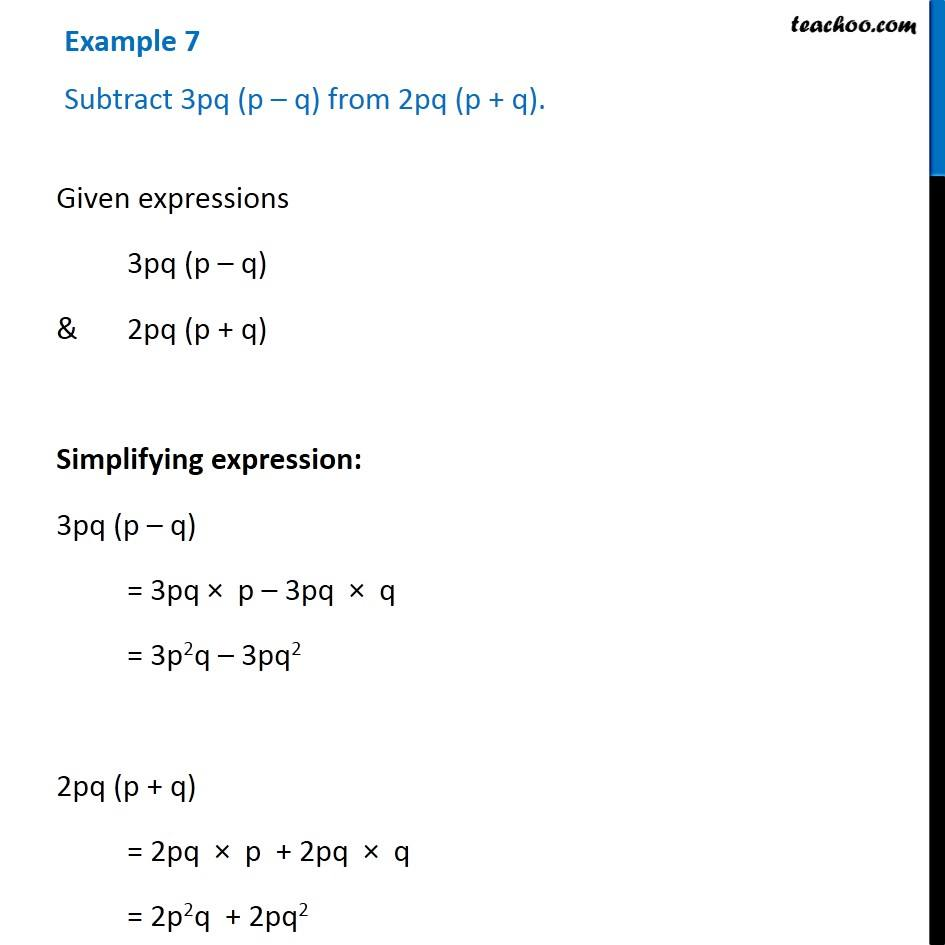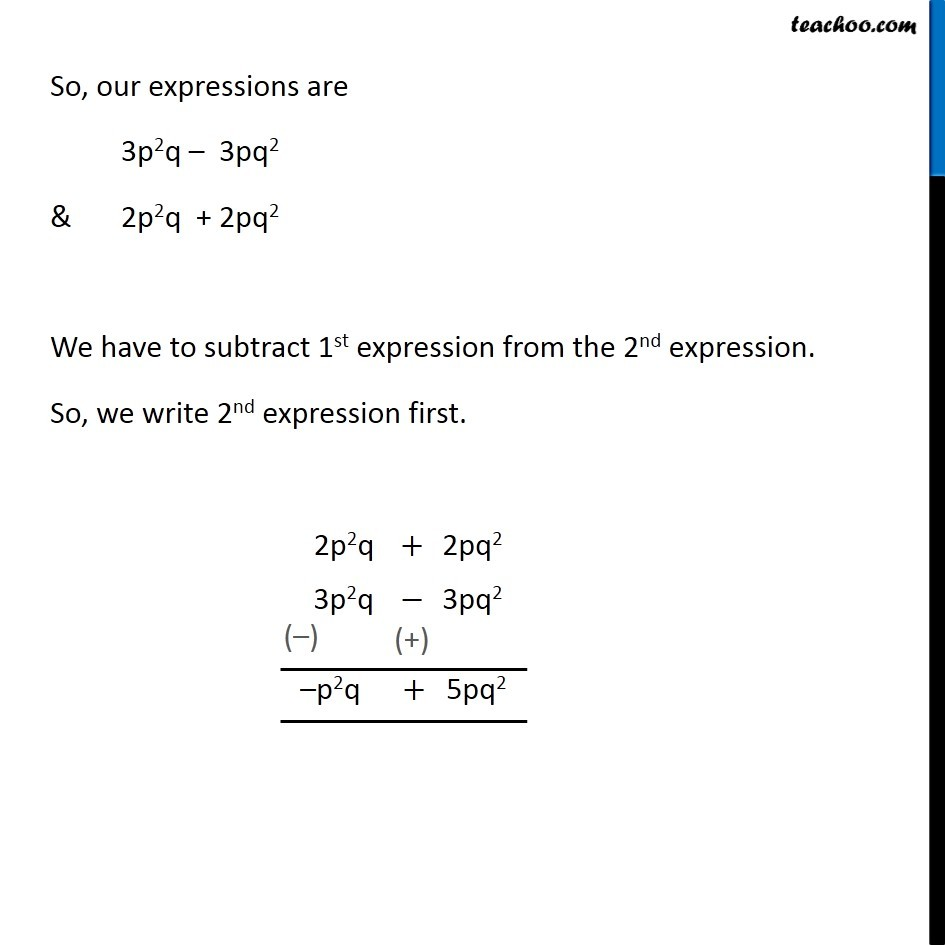Multiplication of Monomials by Binomials and Trinomials

Chapter 8 Class 8 Algebraic Expressions and Identities
Concept wiseLearn in your speed, with individual attention - Teachoo Maths 1-on-1 Class

### Transcript

Example 7 Subtract 3pq (p – q) from 2pq (p + q). Given expressions 3pq (p – q) & 2pq (p + q) Simplifying expression: 3pq (p – q) = 3pq × p – 3pq × q = 3p2q – 3pq2 2pq (p + q) = 2pq × p + 2pq × q = 2p2q + 2pq2 So, our expressions are 3p2q – 3pq2 & 2p2q + 2pq2 We have to subtract 1st expression from the 2nd expression. So, we write 2nd expression first.